# RS Aggarwal Solutions for Class 7 Maths Chapter 19 Three-Dimensional Shapes

RS Aggarwal Solutions for Class 7 Maths Chapter 19 – Three-Dimensional Shapes are available here. Our professional tutors formulate these exercises to help you with your exam preparation to attain good marks in Maths. Students aiming to score good marks in Maths practise RS Aggarwal Class 7 Solutions, which help them to gain more knowledge. This book is one of the top materials when it comes to providing a question bank to practice.

The primary aim is to help students understand and crack these problems. We at BYJU’S have prepared the RS Aggarwal Solutions for Class 7 Maths Chapter 19 wherein problems are solved step by step with detailed explanations. Download pdf of Class 7 Chapter 19 in their respective links.

## Download PDF of RS Aggarwal Solutions for Class 7 Maths Chapter 19 – Three-Dimensional Shapes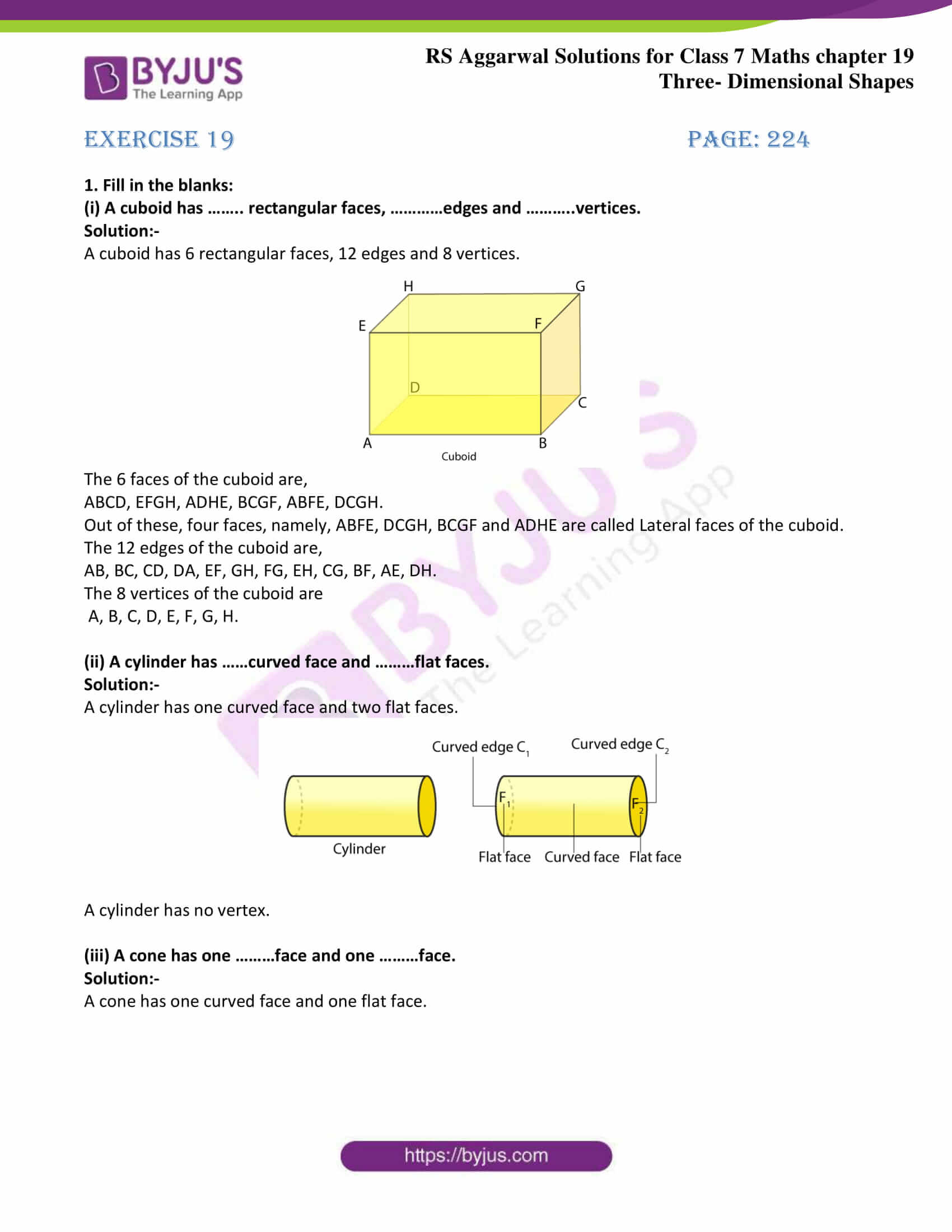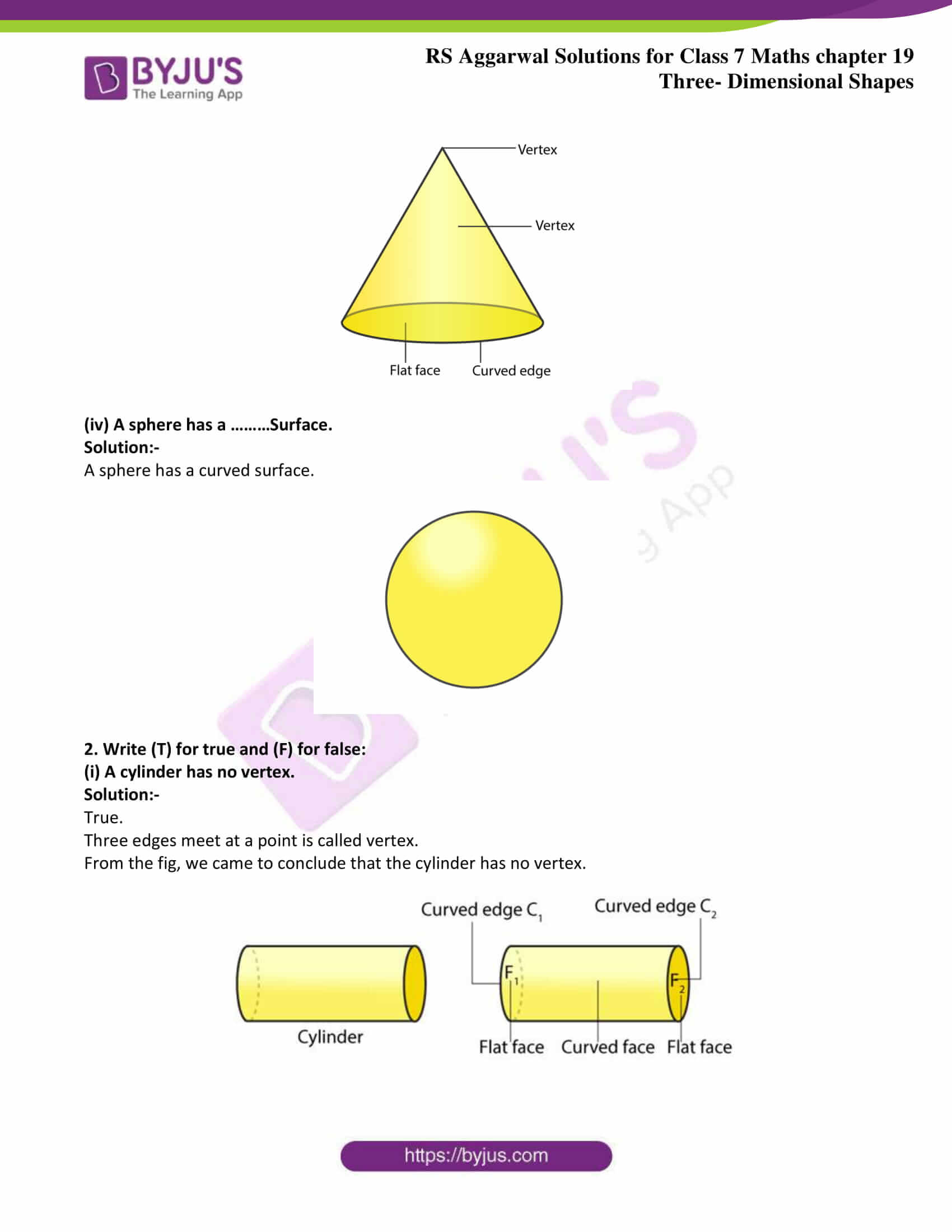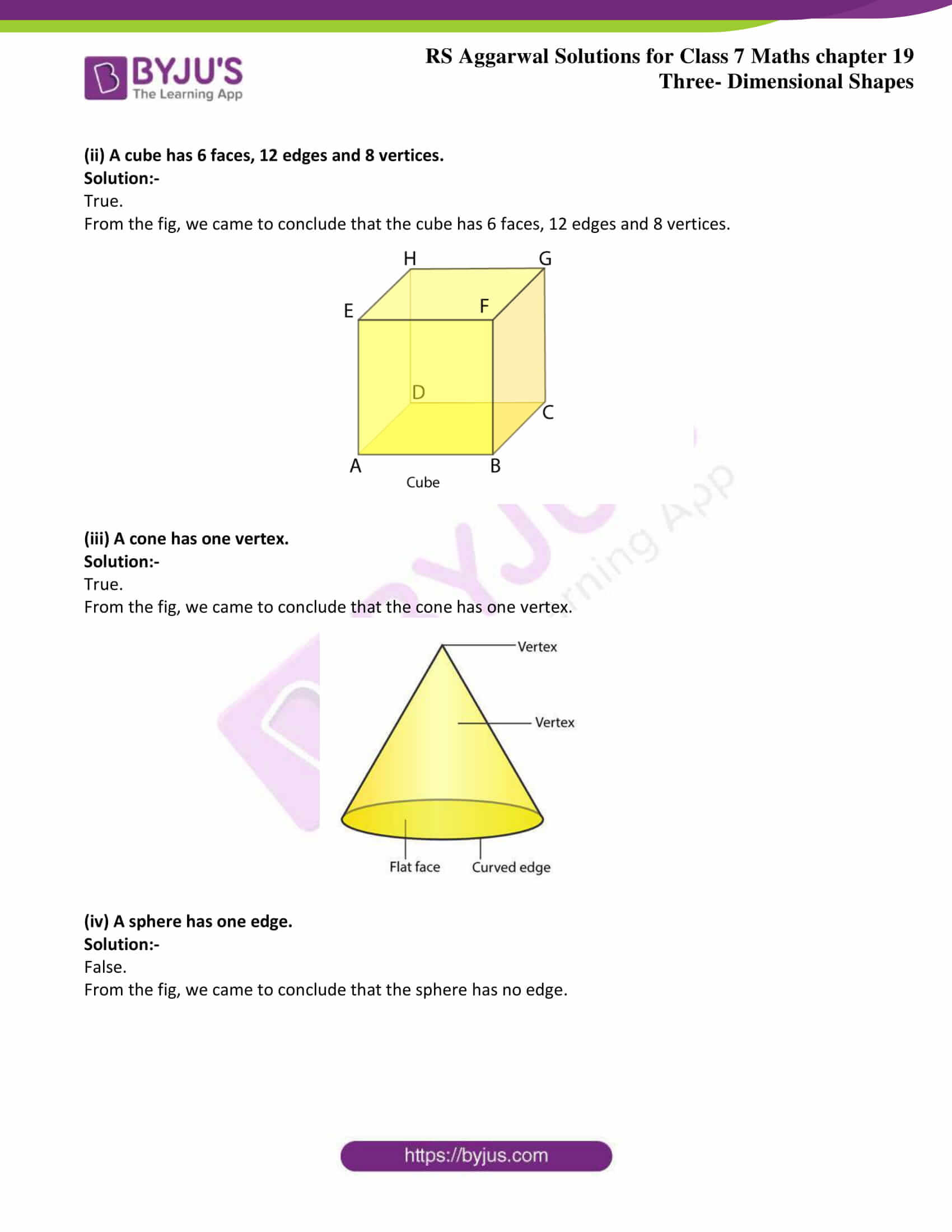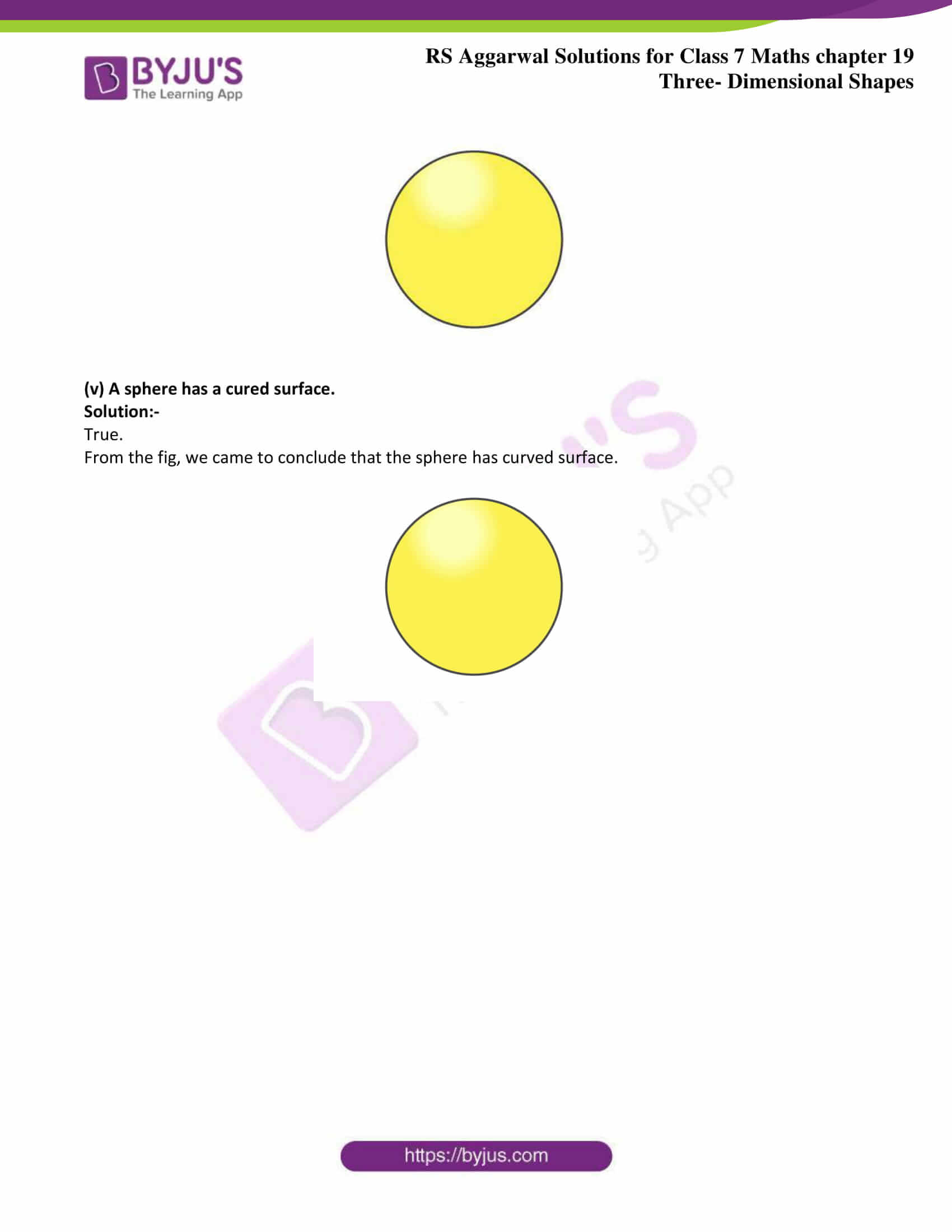## Exercise 19 Page: 224

1. Fill in the blanks:

(i) A cuboid has …….. rectangular faces, …………edges and ………..vertices.

Solution:-

A cuboid has 6 rectangular faces, 12 edges and 8 vertices.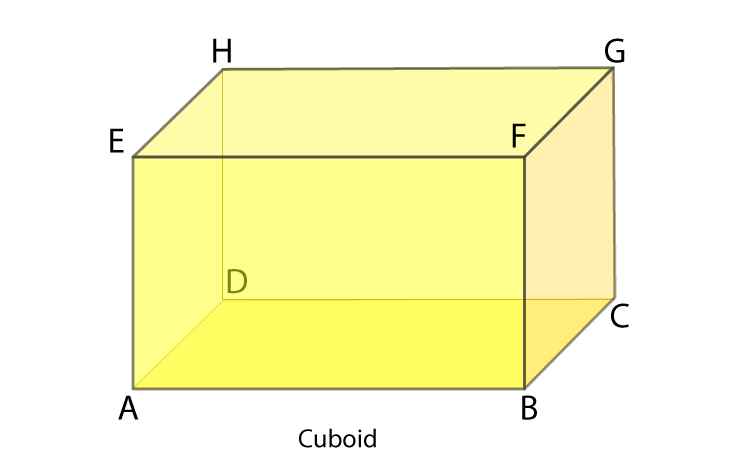The 6 faces of the cuboid are,

ABCD, EFGH, ADHE, BCGF, ABFE, DCGH.

Out of these, four faces, namely, ABFE, DCGH, BCGF and ADHE are called Lateral faces of the cuboid.

The 12 edges of the cuboid are,

AB, BC, CD, DA, EF, GH, FG, EH, CG, BF, AE, DH.

The 8 vertices of the cuboid are

A, B, C, D, E, F, G, H.

(ii) A cylinder has ……curved face and ………flat faces.

Solution:-

A cylinder has one curved face and two flat faces.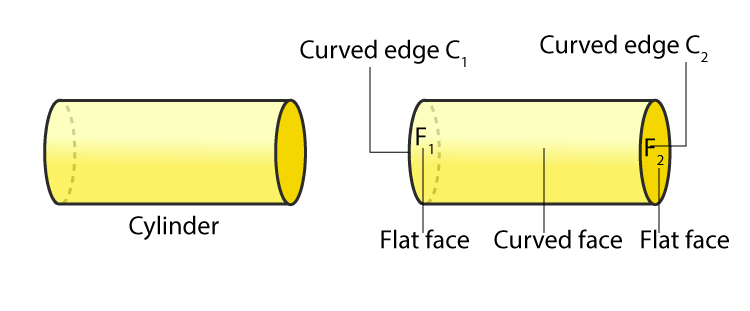A cylinder has no vertex.

(iii) A cone has one ………face and one ………face.

Solution:-

A cone has one curved face and one flat face.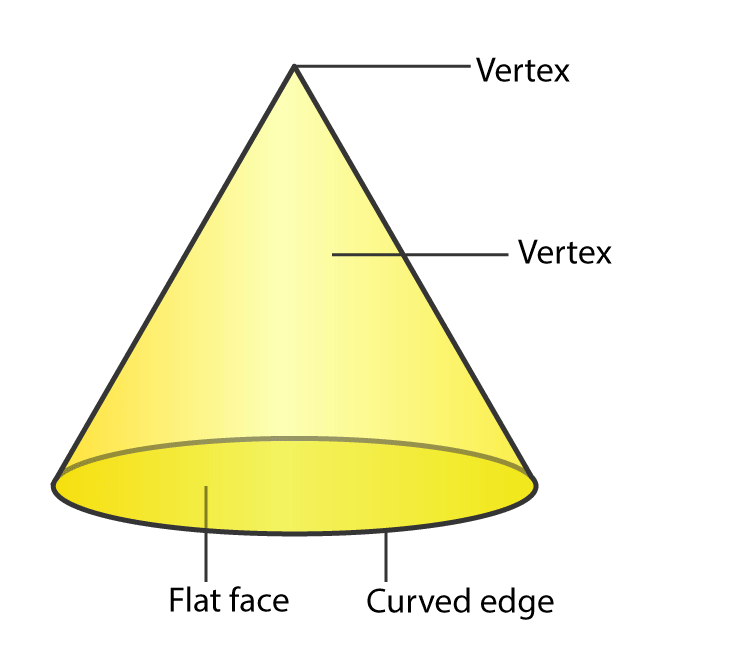(iv) A sphere has a ………Surface.

Solution:-

A sphere has a curved surface.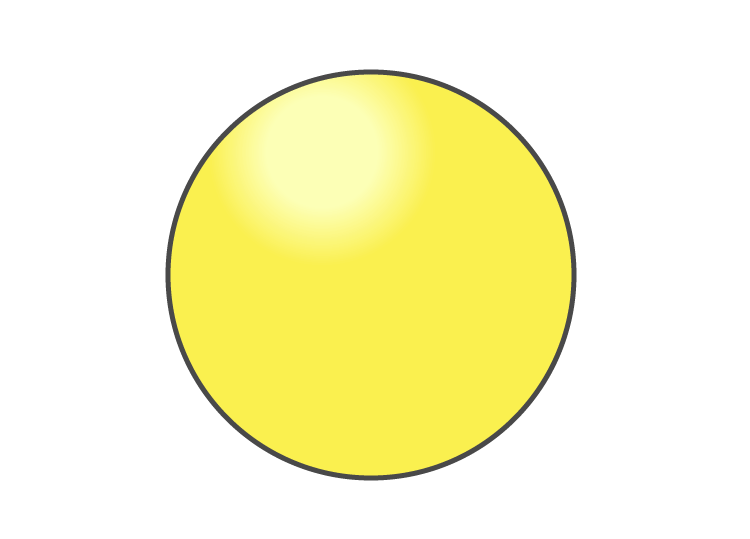2. Write (T) for true and (F) for false:

(i) A cylinder has no vertex.

Solution:-

True.

Three edges meet at a point is called vertex.

From the fig, we came to conclude that the cylinder has no vertex.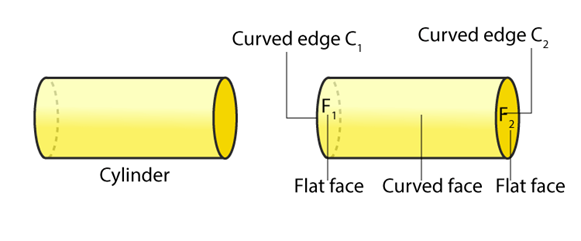(ii) A cube has 6 faces, 12 edges and 8 vertices.

Solution:-

True.

From the fig, we came to conclude that the cube has 6 faces, 12 edges and 8 vertices.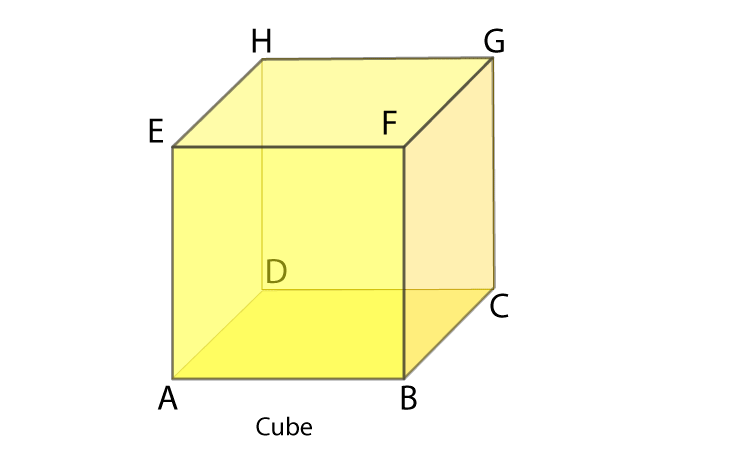(iii) A cone has one vertex.

Solution:-

True.

From the fig, we came to conclude that the cone has one vertex.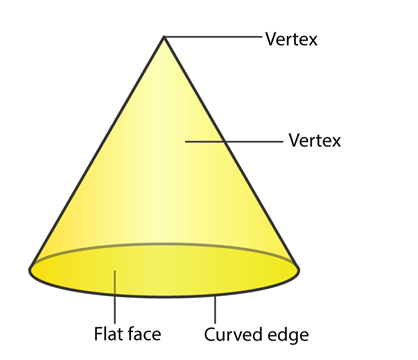(iv) A sphere has one edge.

Solution:-

False.

From the fig, we came to conclude that the sphere has no edge.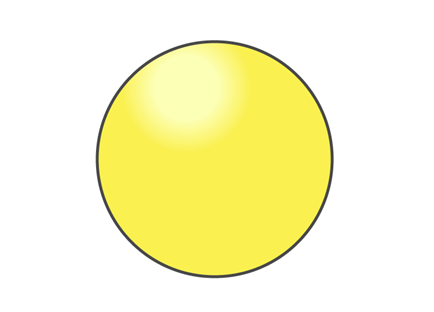(v) A sphere has a cured surface.

Solution:-

True.

From the fig, we came to conclude that the sphere has curved surface.## RS Aggarwal Solutions for Class 7 Maths Chapter 19 – Three-Dimensional Shapes

Chapter 19 – Three-Dimensional Shapes contain 1 exercise and the RS Aggarwal Solutions available on this page provide solutions to the questions present in the exercises. Now, let us have a look at some of the concepts discussed in this chapter.

• Solids
• Cuboid
• Cube
• Cylinder
• Cone
• Sphere
• Net of Three-Dimensional Figure

### Chapter Brief of RS Aggarwal Solutions for Class 7 Maths Chapter 19 – Three-Dimensional Shapes

RS Aggarwal Solutions for Class 7 Maths Chapter 19 – Three-Dimensional Shapes. In this chapter students get to know the various types of solids such as, cuboid, cube, cylinder, cone and sphere. Any figure or any shape which has three dimensions – length, breadth and height is known as a three dimensional shape.